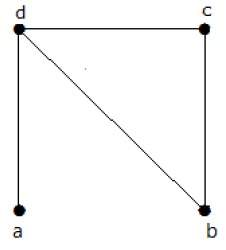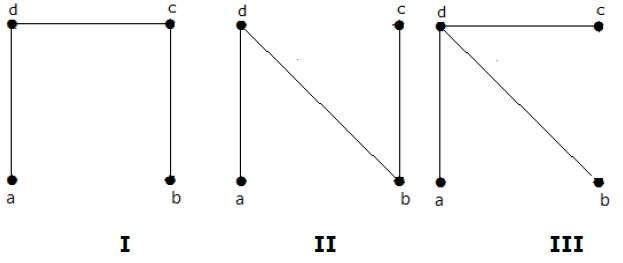# Finding the number of spanning trees in a graph

## Problem Statement

Find the number of spanning trees in the following graph.## Solution

The number of spanning trees obtained from the above graph is 3. They are as follows −These three are the spanning trees for the given graphs. Here the graphs I and II are isomorphic to each other. Clearly, the number of non-isomorphic spanning trees is two.

Updated on: 23-Aug-2019

277 Views